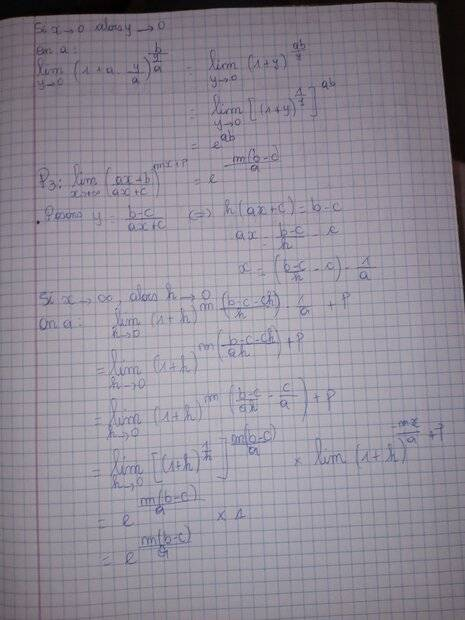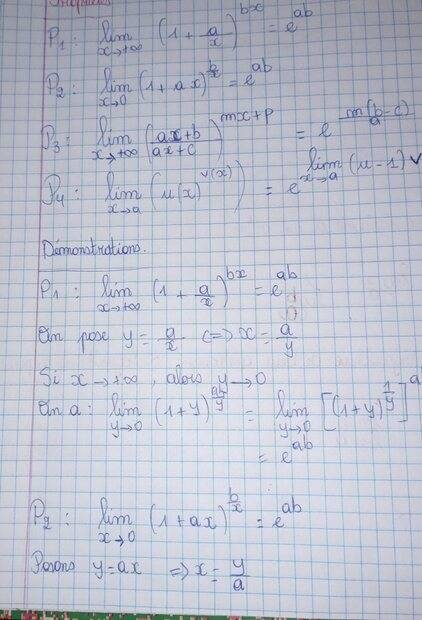# Properties of limits of exponential functions

• A dummy progression
In summary, the conversation is about a proof for P1, where the properties of natural logarithm are used. The speaker also mentions their uncertainty about P4 and requests for clarification regarding the assumptions for u and v in the proof. They also mention the importance of being clear about constants and details in the proof.

#### A dummy progression

Homework Statement
Prove the following properties (in pictures)
Relevant Equations
Using the def. Of natural logarithmI did only the the first three prop.
And on a means we have, on pose or posons means let there be , propriétés means properties, alors meand then.

I apologize i am a french native speaker and i am busy so i cannot rewrite this in entirely english

#### Attachments

I'm not convinced by what you have done for P1. Where did you use the properties of the natural logarithm?

In any case, you need to be clear about what you are assuming in your proof.

My guess is you are allowed to use
$$\lim _{x\to\infty} \left (1+\frac{1}{x} \right )^x = e\tag{1}.$$
For P1, the above implies
$$\lim _{x\to\infty} \left (1+\frac{a}{x} \right )^x = e^{a}$$
and therefore for any ##b\geqslant 0##
$$\lim _{x\to\infty} \left (1+\frac{a}{x} \right )^{bx} = e^{ba}.$$
The others are done similarly. Your task is to manipulate the limit to look like (1).

Be careful with statements like if ##x\to 0##, then ##y:= \frac{a}{x}\to \infty\ (a>0)##. This is true assuming ##x\to 0+##. It's clear ##a,b## are some fixed constants, but specify whether they are negative/non-negative. These details are important.

Last edited:
•PeroK
PeroK said:
I'm not convinced by what you have done for P1. Where did you use the properties of the natural logarithm?

In any case, you need to be clear about what you are assuming in your proof.
I don't know how to do P4. But i proved all of the first three

nuuskur said:
My guess is you are allowed to use
$$\lim _{x\to\infty} \left (1+\frac{1}{x} \right )^x = e\tag{1}.$$For P1, the above implies
$$\lim _{x\to\infty} \left (1+\frac{a}{x} \right )^x = e^{a}$$
and therefore for any ##b\geqslant 0##
$$\lim _{x\to\infty} \left (1+\frac{a}{x} \right )^{bx} = e^{ba}.$$
The others are done similarly. Your task is to manipulate the limit to look like (1).

Be careful with statements like if ##x\to 0##, then ##y:= \frac{a}{x}\to \infty\ (a>0)##. This is true assuming ##x\to 0+##. It's clear ##a,b## are some fixed constants, but specify whether they are negative/non-negative. These details are important.
On pose : we set , on a : we have , alors : then.

I didn't find P4 . Could you explain it to me?

What is assumed of ##u## and ##v##? Details are important! Do you seek to prove
$$\lim _{x\to a} u(x) ^{v(x)} = \exp \left ( \lim _{x\to a} (u(x)-1)v(x) \right )?$$
If so, then don't bother, for this is false.

It is true that
$$\lim _{x\to a} u(x) ^{v(x)} = u(a) ^{v(a)}$$
assuming ##u(x)^{v(x)}## is well defined around ##a## and ##u,v## are continuous at ##a##.